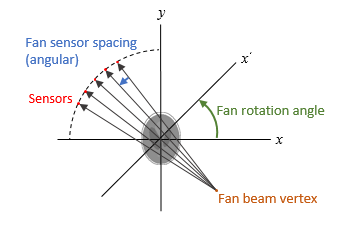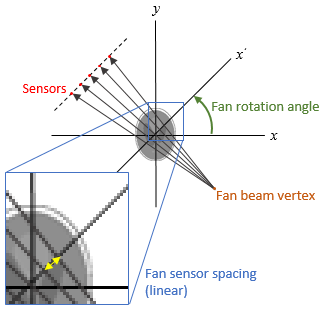Documentation

This is machine translation

Mouseover text to see original. Click the button below to return to the English version of the page.

para2fan

Convert parallel-beam projections to fan-beam

Syntax

F = para2fan(P,D)
F = fan2para(P,D,Name,Value)
[F,fan_sensor_positions,fan_rotation_angles] = fan2para(___)

Description

example

F = para2fan(P,D) converts the parallel-beam data P to the fan-beam data F. Each column of P contains the parallel-beam sensor samples at one rotation angle. D is the distance from the fan-beam vertex to the center of rotation.The parallel-beam sensors are assumed to have a one-pixel spacing. The parallel-beam rotation angles are spaced equally to cover [0,180] degrees. The calculated fan-beam rotation angles have the same spacing as the parallel-beam rotation angles, and cover [0,360) degrees. The calculated fan-beam angles are equally spaced with the spacing set to the smallest angle implied by the sensor spacing.
F = fan2para(P,D,Name,Value) uses name-value pairs to control aspects of the data conversion. Argument names can be abbreviated, and case does not matter.
[F,fan_sensor_positions,fan_rotation_angles] = fan2para(___) returns the fan-beam sensor locations in fan_sensor_positions and rotation angles in fan_rotation_angles.

Examples

collapse all

Generate parallel-beam projections

ph = phantom(128); theta = 0:180; [P,xp] = radon(ph,theta); imshow(P,[],'XData',theta,'YData',xp,'InitialMagnification','fit') axis normal title('Parallel-Beam Projections') xlabel('\theta (degrees)') ylabel('x''') colormap(gca,hot), colorbarConvert to fan-beam projections

[F,Fpos,Fangles] = para2fan(P,100); figure imshow(F,[],'XData',Fangles,'YData',Fpos,'InitialMagnification','fit') axis normal title('Fan-Beam Projections') xlabel('\theta (degrees)') ylabel('Sensor Locations (degrees)') colormap(gca,hot), colorbarInput Arguments

collapse all

Parallel-beam projection data, specified as a numeric matrix. Each column of P contains the parallel-beam data at one rotation angle. The number of columns indicates the number of parallel-beam rotation angles and the number of rows indicates the number of parallel-beam sensors.

Data Types: double | single

Distance in pixels from the fan beam vertex to the center of rotation, specified as a positive number. para2fan assumes that the center of rotation is the center point of the projections, which is defined as ceil(size(F,1)/2). The value of D must be greater than or equal to ParallelSensorSpacing*(SIZE(P,1)-1)/2

The figure illustrates D in relation to the fan-beam vertex for one fan-beam projection.Data Types: double | single

Name-Value Pair Arguments

Specify optional comma-separated pairs of Name,Value arguments. Name is the argument name and Value is the corresponding value. Name must appear inside quotes. You can specify several name and value pair arguments in any order as Name1,Value1,...,NameN,ValueN.

Example: F = para2fan(P,D,'FanRotationIncrement',5)

Range of fan-beam rotation, specified as the comma-separated pair consisting of 'FanCoverage' and one of these values.

• 'cycle' — Rotate through the full range [0, 360) degrees.

• 'minimal' — Rotate through the minimum range necessary to represent the object.

Fan-beam rotation angle increment in degrees, specified as the comma-separated pair consisting of 'FanRotationIncrement' and a positive scalar.

• If FanCoverage is 'cycle', then 360/FanRotationIncrement must be an integer.

• If you do not specify FanRotationIncrement, then the default value is equal to the parallel-beam rotation angle.Data Types: double

Fan-beam sensor positioning, specified as the comma-separated pair consisting of 'FanSensorGeometry' and one of the following values.

Value

Meaning

Diagram

'arc'

Sensors are spaced at equal angles along a circular arc at distance D from the center of rotation.

FanSensorSpacing defines the angular spacing in degrees.'line'

Sensors are spaced at equal distances along a line that is parallel to the x' axis. The closest sensor is distance D from the center of rotation.

FanSensorSpacing defines the distance between fan-beams on the x' axis, in pixels.Fan-beam sensor spacing, specified as the comma-separated pair consisting of 'FanSensorSpacing' and a positive scalar.

• If FanSensorGeometry is 'arc', then FanSensorSpacing defines the angular spacing in degrees.

• If FanSensorGeometry is 'line', then FanSensorSpacing defines the linear distance between fan-beams, in pixels. Linear spacing is measured on the x' axis.

If you do not specify FanSensorGeometry, then the default value of FanSensorSpacing is the smallest value implied by ParallelSensorSpacing such that:

• If FanSensorGeometry is 'arc', then FanSensorSpacing is 180/pi*ASIN(ParallelSensorSpacing/D)

• If FanSensorGeometry is 'line', then FanSensorSpacing is D*ASIN(ParallelSensorSpacing/D)

Data Types: double

Type of interpolation used between the parallel-beam and fan-beam data, specified as the comma-separated pair consisting of 'Interpolation' and one of these values.

'nearest' — Nearest-neighbor

'linear' — Linear (the default)

'spline' — Piecewise cubic spline

'pchip' — Piecewise cubic Hermite (PCHIP)

Range of parallel-beam rotation, specified as the comma-separated pair consisting of 'ParallelCoverage' and one of these values.

• 'cycle' — Parallel data covers the full range of [0, 360) degrees.

• 'halfcycle' — Parallel data covers [0, 180) degrees.

Parallel-beam sensor spacing in pixels, specified as the comma-separated pair consisting of 'ParallelSensorSpacing' and a positive scalar.

Data Types: double

Output Arguments

collapse all

Fan-beam projection data, returned as a numeric matrix. Each column of F contains the fan-beam sensor samples at one rotation angle.

Parallel-beam projection data, returned as a numeric matrix. Each column of F contains the fan-beam data at one rotation angle. The number of columns indicates the total number of fan-beam rotation angles and is equal to the length of fan_rotation_angles. The number of rows indicates the total number of parallel-beam sensors and is equal to the length of fan_sensor_positions.

Data Types: double

Fan-beam sensor locations, returned as a numeric column vector.

• If 'FanSensorGeometry' is 'arc' (the default), then fan_sensor_positions contains the fan-beam sensor measurement angles.

• If 'FanSensorGeometry' is 'line', then fan_sensor_positions contains the fan-beam sensor positions along the line of sensors.

Data Types: double

Fan-beam rotation angles, returned as a numeric row vector.

Data Types: double